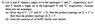# Comparing product topologies

• UJS

#### UJS

I'm reading Topology by Munkres, and I'm having some trouble with exercise 5 on page 92 (screenshot attached).

## Homework Statement

Let X and X' denote a single set in the topologies T and T', respectively; let Y and Y' denote a single set in the topologies U and U', respectively. Assume these sets are nonempty.
Show that if T' is finer than T and U' is finer than U, then the product topology on X' x Y' is finer than the product topology on X x Y.

## The Attempt at a Solution

I think this is a counterexample...
Let T = T' = U = U' be the standard topology on ℝ. Let X = Y = Y' = (0, 1). Let X' = (2, 3).

X is open in X and Y is open in Y → X x Y is open in X x Y.
X is not a subset of X' →X is not open in X' → X x Y is not open in X' x Y'.
Hence the product topology on X' x Y' is not finer than the product topology on X x Y.

#### Attachments

•topology.jpg
27.4 KB · Views: 383